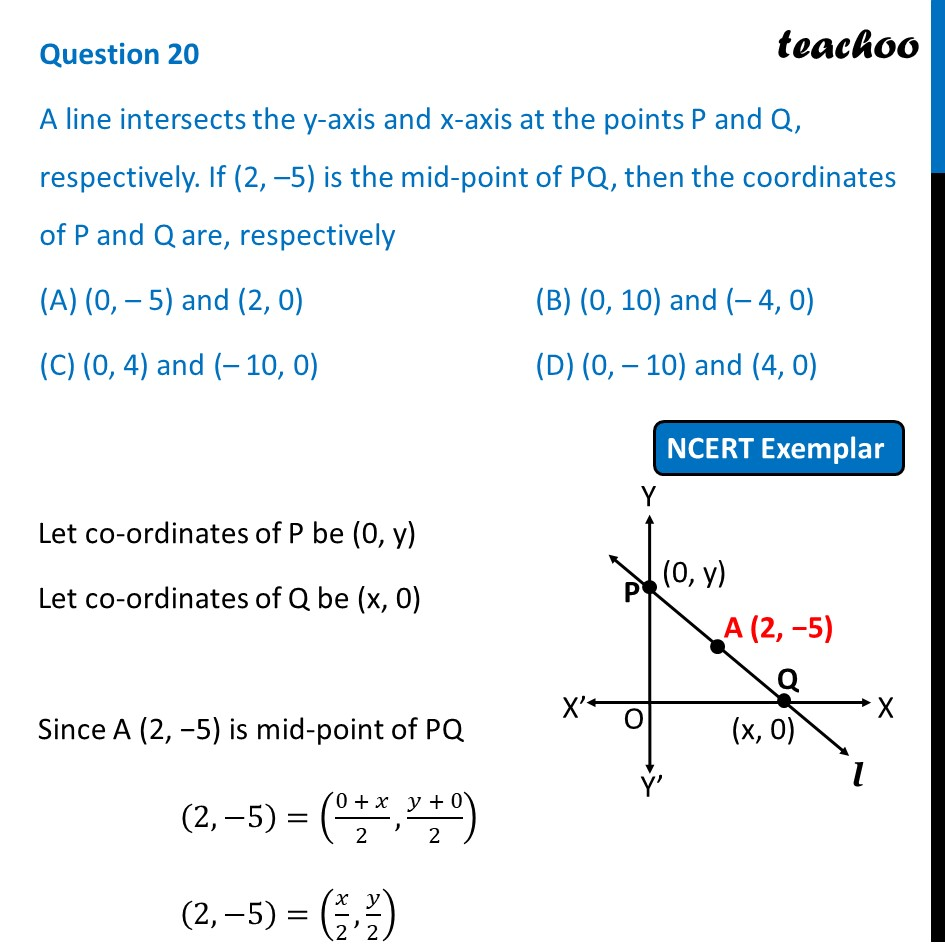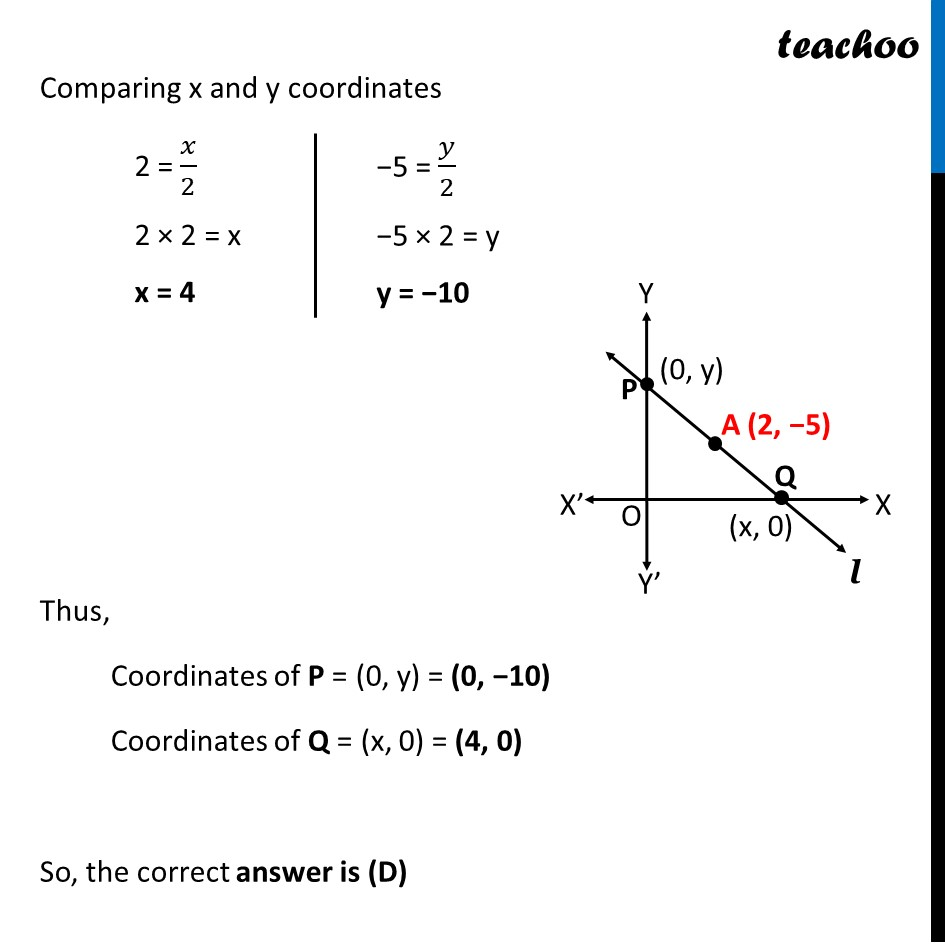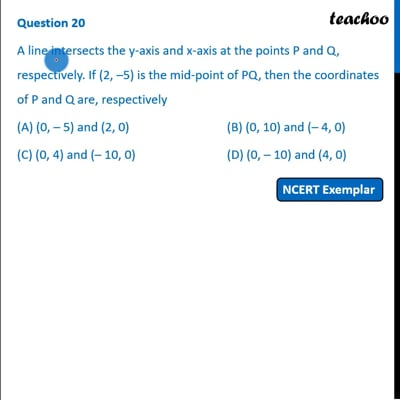NCERT Exemplar - MCQ

Chapter 7 Class 10 Coordinate Geometry
Serial order wise

## (D) (0, – 10) and (4, 0)This video is only available for Teachoo black users

Introducing your new favourite teacher - Teachoo Black, at only ₹83 per month

### Transcript

Question 20 A line intersects the y-axis and x-axis at the points P and Q, respectively. If (2, –5) is the mid-point of PQ, then the coordinates of P and Q are, respectively (A) (0, – 5) and (2, 0) (B) (0, 10) and (– 4, 0) (C) (0, 4) and (– 10, 0) (D) (0, – 10) and (4, 0) Let co-ordinates of P be (0, y) Let co-ordinates of Q be (x, 0) Since A (2, −5) is mid-point of PQ (2,−5)=((0 + 𝑥)/2,(𝑦 + 0)/2) (2,−5)=(𝑥/2,𝑦/2) Comparing x and y coordinates 2 = 𝑥/2 2 × 2 = x x = 4 −5 = 𝑦/2 −5 × 2 = y y = −10 Thus, Coordinates of P = (0, y) = (0, −10) Coordinates of Q = (x, 0) = (4, 0) So, the correct answer is (D)